This is the rendered collections of notes by me, Lex Toumbourou.

You can find the source on the GitHub project.

The notes are collecting using my interpretation of the Zettelkasten method.

More
• ## Eigenvector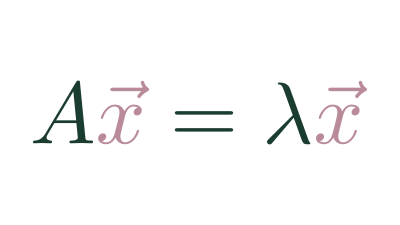A set of vectors whose span doesn't change after a transformation.

• ## Changing Basis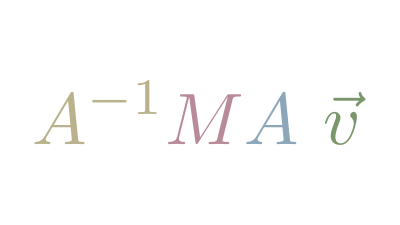Since any vectors can be Basis Vectors, it's useful to understand how to translate vectors between bases

• ## Roblox Attachment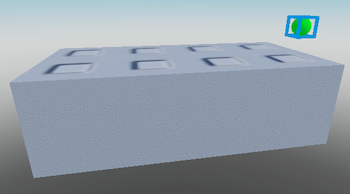An object that describes a point and orientation relative to a BasePart

• ## Cross Product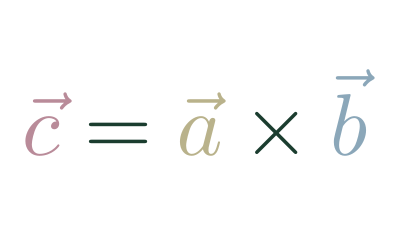An operation between two 3d vectors that returns a vector.

• ## Matrix Determinate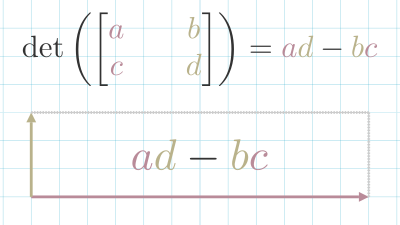A measure of how a matrix scales space.

• ## Basis Vectors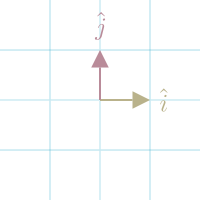The set of vectors that defines space.

• ## Roblox CFrame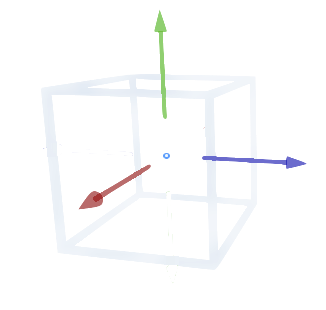In Roblox, a CFrame (coordinate frame) is an object that encodes position and rotation in 3D space.

• ## Identity Matrix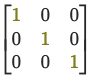When you multiply a matrix $(A)$ by the Identity Matrix $(I)$, you get the original matrix back.

$A …$